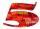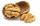Seamstress 2

Seamstress bought two kinds of textile in whole meters. One at 50 SKK and the second 70 SKK per meter. How many meter bought from this two textiles when paid totally 1540 SKK?

Result

x =  7 m
y =  17 m

Solution:Leave us a comment of this math problem and its solution (i.e. if it is still somewhat unclear...):Be the first to comment!To solve this verbal math problem are needed these knowledge from mathematics:

Do you want to calculate least common multiple two or more numbers?

Next similar examples:

1. Factory and divisionsThe factory consists of three auxiliary divisions total 2,406 employees. The second division has 76 employees less than 1st division and 3rd division has 212 employees more than the 2nd. How many employees has each division?
2. Theorem proveWe want to prove the sentence: If the natural number n is divisible by six, then n is divisible by three. From what assumption we started?
3. NumbersWrite smallest three-digit number, which in division 5 and 7 gives the rest 2.
4. Nineteenth memberFind the nineteenth member of the arithmetic sequence: a1=33 d=5 find a19
5. One halfOne half of ? is: ?
6. Functions f,gFind g(1) if g(x) = 3x - x2 Find f(5) if f(x) = x + 1/2
7. Two equationsSolve equations (use adding and subtracting of linear equations): -4x+11y=5 6x-11y=-5
8. Linear systemSolve a set of two equations of two unknowns: 1.5x+1.2y=0.6 0.8x-0.2y=2
9. Volleyball8 girls wants to play volleyball against boys. On the field at one time can be six players per team. How many initial teams of this girls may trainer to choose?
10. Divisible by 5How many three-digit odd numbers divisible by 5, which are in place ten's number 3?
11. PowersExpress the expression ? as the n-th power of the base 10.
12. Percentage increaseIncrease number 400 by 3.5%
13. First manWhat is the likelihood of a random event where are five men and seven women first will leave the man?
14. 6 termsFind the first six terms of the sequence. a1 = 7, an = an-1 + 6
15. TreesA certain species of tree grows an average of 0.5 cm per week. Write an equation for the sequence that represents the weekly height of this tree in centimeters if the measurements begin when the tree is 200 centimeters tall.
16. NutsHow many we must have at least nuts if we can equally divide it to 10 children, 11 children or 19 children and any nut left?
17. Sequence 2Write the first 5 members of an arithmetic sequence a11=-14, d=-1